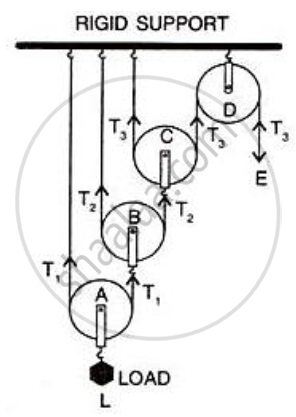Share
Notifications

View all notifications
Books Shortlist
Your shortlist is empty

# Draw a Diagram of Combination of Three Movable Pulleys with a Fixed Pulley Showing the Directions of Load, Effort and Tension in Each Strand. Find:: (I) Mechanical Advantage,(Ii) Velocity Ration and - ICSE Class 10 - Physics

Login
Create free account

Forgot password?

#### Question

Draw a diagram of combination of three movable pulleys with a fixed pulley showing the directions of load, effort and tension in each strand. Find: (i) mechanical advantage, (ii) Velocity ration and (iii) efficiency of the combination in ideal situation.

#### Solution

Diagram :Tension T1 in the string passing over the pulley A is given as
2T1 = L or T1 = L/2
Tension T2 in the string passing over the pulley B is given as
2T2 = T1or T2 = T1/2 = L/22
Tension T3 in the string passing over the pulley C is given as
2T3 = T2 or T3 = T2/2 = L/23
In equilibrium, T3 = E
E = L/23

Mechanical advantage = MA = L/E = 2^3
As one end of each string passing over a movable pulley is fixed, so the free end of string moves twice the distance moved by the movable pulley.
If load L moves up by a distance x, dL = x, effort moves by a distance 2^3
x, dE = 2^3x

Velocity Ration VR = "Distance moved by the effort dE "/"Distance moved by the load dL " = (2^3 x)/x= 2^3

Efficiency = MA/VR =2^3/2^3 = 1 OR 100%

Is there an error in this question or solution?

#### APPEARS IN

Solution Draw a Diagram of Combination of Three Movable Pulleys with a Fixed Pulley Showing the Directions of Load, Effort and Tension in Each Strand. Find:: (I) Mechanical Advantage,(Ii) Velocity Ration and Concept: Concept of Pulley System.
S